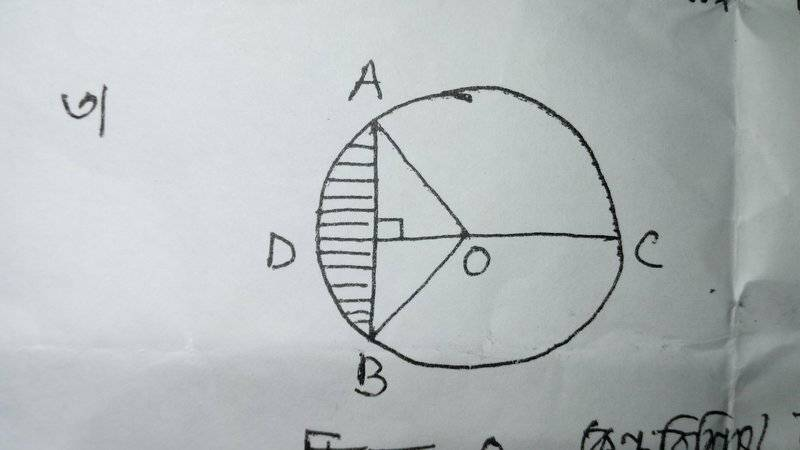# Circle Problem

Member warned that the homework template must be used.
Known data:In the picture, CD = 10 cm. What is the area of shaded area?

Equation:
I know,
Area of a circle = ##πr^2##
Diameter of a circle = ##2πr##

Attempt:
Here, ## r = 10/2 = 5 ##
So, diameter ## = 2πr = 2*3.1416*5 = 31.42 cm ##
And area of the circle ## = πr^2 = 3.1416*5^2 = 78.54 cm^2 ##
But I don't know how to find out the area of sheded section.

#### Attachments

Last edited:

Mark44
Mentor
View attachment 226609
In the picture, CD = 10 cm. What is the area of shaded area?

I know,
Area of a circle = ##πr^2##
Diameter of a circle = ##2πr##

Here, ## r = 10/2 = 5 ##
So, diameter ## = 2πr = 2*3.1416*5 = 31.42 cm ##
And area of the circle ## = πr^2 = 3.1416*5^2 = 78.54 cm^2 ##
But I don't know how to find out the area of sheded section.
You don't have enough information to get a numerical value for this area, as it depends on the angle between the two rays that define the circle sector. The best you can do is to get an expression that involves this angle.

•You don't have enough information to get a numerical value for this area, as it depends on the angle between the two rays that define the circle sector. The best you can do is to get an expression that involves this angle.
Can I get the value of AB?

Mark44
Mentor
Can I get the value of AB?
No, not without knowing that angle. You can write an expression that involves the angle, but you can't get a numerical value without knowing the angle.

•No, not without knowing that angle. You can write an expression that involves the angle, but you can't get a numerical value without knowing the angle.
It means it is an unanswerable question. Isn't it? It is a question from creative talent hunt.

Ray Vickson
Homework Helper
Dearly Missed
It means it is an unanswerable question. Isn't it? It is a question from creative talent hunt.

Perhaps they want you to supply some additional information, or create a question of your own! For example, if you let ##x## be the length of segment AB, you can try to develop formulas for the shaded area and the angle DOA in terms of ##x##. Alternatively, you can assume that ##y## is the angle DOA and then try to develop a formula for ##x## or for the shaded area.

•LCKurtz
Homework Helper
Gold Member
Or maybe they were hoping you would give the range of possible values for the shaded area. You could give its min and max possible values quickly without solving for the area in terms of the angle.

•Or maybe they were hoping you would give the range of possible values for the shaded area. You could give its min and max possible values quickly without solving for the area in terms of the angle.
But how? Would you give me hint?

LCKurtz
Homework Helper
Gold Member
But how? Would you give me hint?
Try drawing the figure with angle AOB smaller. A lot smaller. Then larger. How small and large can it get? What happens to the shaded area when you do it?

•Try drawing the figure with angle AOB smaller. A lot smaller. Then larger. How small and large can it get? What happens to the shaded area when you do it?
0° to 360°
0 sq. cm to 78.54 sq. cm
Right or not?

LCKurtz
Homework Helper
Gold Member
Right.

•Ray Vickson
Homework Helper
Dearly Missed
Known data:
View attachment 226609
In the picture, CD = 10 cm. What is the area of shaded area?

Equation:
I know,
Area of a circle = ##πr^2##
Diameter of a circle = ##2πr##

Attempt:
Here, ## r = 10/2 = 5 ##
So, diameter ## = 2πr = 2*3.1416*5 = 31.42 cm ##
And area of the circle ## = πr^2 = 3.1416*5^2 = 78.54 cm^2 ##
But I don't know how to find out the area of sheded section.
•